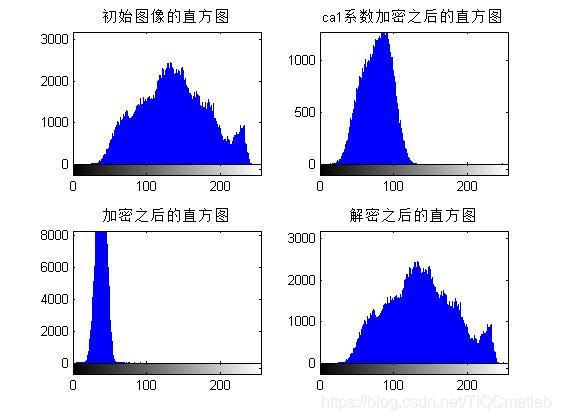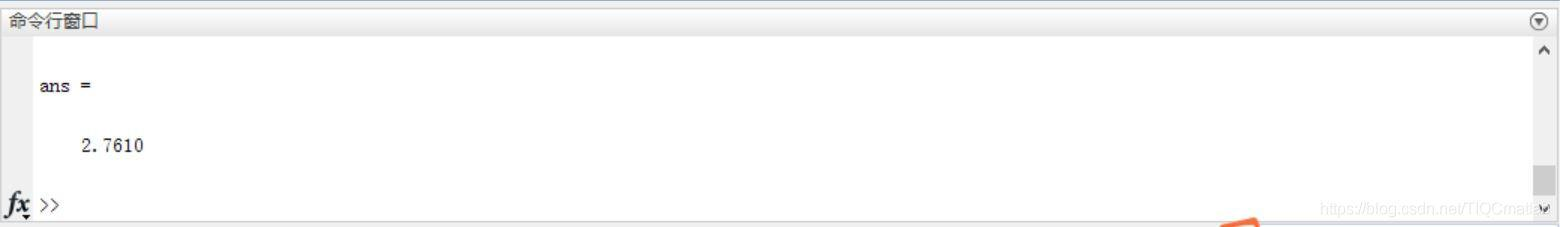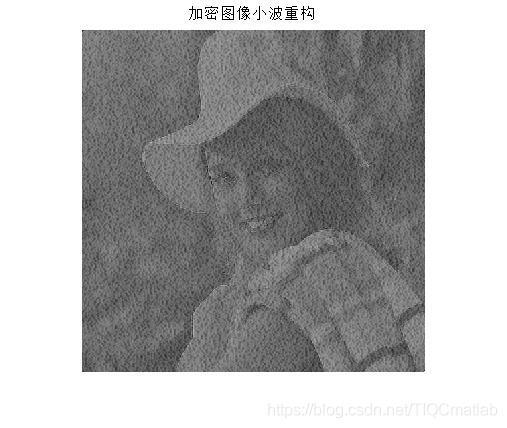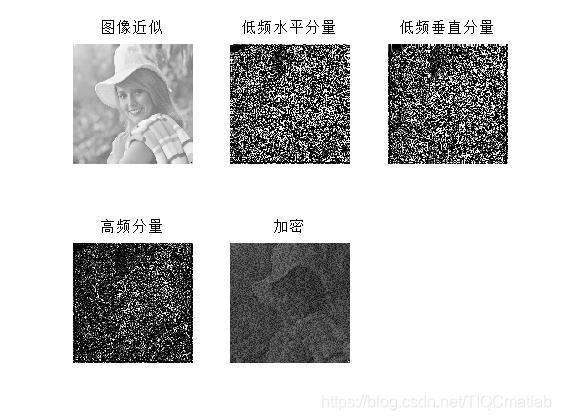# 【影象加密】基於matlab混沌演算法影象加密解密【含Matlab原始碼 1218期】

## 二、部分原始碼

```c clear all; t0 = clock;%測試程式執行時間 im=imread('elain.jpg'); im1=rgb2gray(im);%影象灰度化 im1=medfilt2(im1,[3 3]);%影象平滑處理 subplot(331); figure(1); imshow(im);%X為原始影象 title('原始影象'); figure(2); imshow(im1); title('灰度化處理'); im1=double(im1); [ca1,ch1,cv1,cd1]=dwt2(im1,'bior3.7');%小波變換，獲取影象的低頻高頻係數 figure(3); subplot(231); imshow(ca1,[]); title('影象近似'); subplot(232); imshow(ch1); title('低頻水平分量'); subplot(233); imshow(cv1); title('低頻垂直分量'); subplot(234); imshow(cd1),; title('高頻分量'); %以下為混沌加密演算法 %以下為混沌加密演算法 %以下為混沌加密演算法 %以下為混沌加密演算法 %以下為混沌加密演算法 %以下為混沌加密演算法 [M,N]=size(ca1); e=hundungen555(M,N,0.1); tt=0.1; fca1=mod(ttca1+(1-tt)e,256); subplot(235); imshow(fca1,[]); title('加密'); im2=idwt2(ca1,ch1,cv1,cd1,'bior3.7'); figure(4); imshow(uint8(im2),[]); title('灰度影象小波重構'); im3=idwt2(fca1,ch1,cv1,cd1,'bior3.7'); figure(5); imshow(uint8(im3),[]); title('加密影象小波重構'); %以下為混沌解密演算法 %以下為混沌解密演算法 %以下為混沌解密演算法 %以下為混沌解密演算法 %以下為混沌解密演算法 %以下為混沌解密演算法 function e=hundungen(M,N,key0) key0=3.925key0(1-key0); end key1=3.925; for(i=1:M) for(j=1:N) key0=key1key0(1-key0); a(i,j)=key0; end end key3=0.2; key2=3.925; for(i=1:M) for(j=1:N) key3=key2key3(1-key3); b(i,j)=key3; end end key4=0.3; key2=3.925; for(i=1:M) for(j=1:N) key4=key2key4(1-key4); c(i,j)=key4; end end t=0.4; w0=0.2; w1=0.5; w2=0.3; w=(1-t)^2w0+2t(1-t)w1+t^2w2; for(i=1:M) for(j=1:N)
P(i,j)=(1-t)^2
a(i,j)w0+2t(1-t)b(i,j)w1+t^2c(i,j)*w2; d(i,j)=P(i,j)/w; d(i,j)=P(i,j); end end x=d;

end

```

## 三、執行結果## 四、matlab版本及參考文獻

1 matlab版本 2014a

2 參考文獻  蔡利梅.MATLAB影象處理——理論、演算法與例項分析[M].清華大學出版社，2020. 楊丹,趙海濱,龍哲.MATLAB影象處理例項詳解[M].清華大學出版社，2013. 周品.MATLAB影象處理與圖形使用者介面設計[M].清華大學出版社，2013. 劉成龍.精通MATLAB影象處理[M].清華大學出版社，2015.